# NCRT Solutions Excercise 1

Question 1: Find the radian measures corresponding to the following degree measures:

(i) 25° (ii) – 47° 30' (iii) 240° (iv) 520°

As we know  180° = π radian

(ii)  –47° 30'

–47° 30'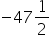=-95/2 degree

(iii)  240°

We know that 180° = π radian

Question 2: Find the degree measures corresponding to the following radian measures

(Use π =22/7)

(i) 11/16  (ii)   – 4 (iii)    5π/3 (iv)  7π/6

As we know that π radian = 180°

Therefore 11/16 radian=180/ π x 11/16 degree

= 45 x 11/ π x 4 degree

=45 x 11 x 7/ 22 x 4 degree

=315/8 degree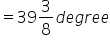=39o+3 x 60/8 minutes                 [1o=60’]

=39o + 22’ + ½ minutes

=39o22’30”                          [1’=60]

(ii)   – 4

We know that π radian = 180°

-4 radian=180/π x (-4) degree=180 x 7(-4)/22 degree

=-2520/11 degree=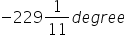=-229o+1 x 60/11 minutes            [1o=60’]

=-229o+5’+5/11 minutes

=-229o5’27”                                                [1’=60”]

(iii)  5 π/3

We know that π radian = 180°

5 π/3 radian=180/π x 5 π/3 degree=300o

(iv)  7 π /6

We know that π radian = 180°

∴ 7 π /6 radian=180/π x 7 π/6 =210o

Question 3: A wheel makes 360 revolutions in one minute. Through how many radians does it turn in one second?

Answer 3: Number of revolutions made by the wheel in 1 minute = 360

∴ Number of revolutions made by the wheel in 1 second =360/60=6

In one complete revolution, the wheel turns through an angle of 2π radian.

Hence, in 6 complete revolutions, it will turn through an angle of 6 × 2π radian, i.e., 12 π radian

Thus, in one second, the wheel turns an angle of 12π radian.

Question 4: Find the degree measure of the angle subtended at the centre of a circle of radius 100 cm by an arc of length 22 cm.

(Use π =22/7)

Answer 4: We know that in a circle of radius r unit, if an arc of length l unit subtends an angle θ radian at the centre, then

θ =1/r

Therefore, for= 100 cm, l = 22 cm, we have

θ =22/100 radian=180/π x 22/100 degree=180 x 7 x 22/22 x 100 degree

=126/10 degree=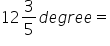12o36’                     [1o=60]

Thus, the required angle is 12°36′.

Question 5: In a circle of diameter 40 cm, the length of a chord is 20 cm. Find the length of minor arc of the chord.

Answer 5: Diameter of the circle = 40 cm

∴Radius (r) of the circle =40/2 cm=20 cm

Let AB be a chord (length = 20 cm) of the circle.

In âˆ†OAB, OA = OB = Radius of circle = 20 cm

Also, AB = 20 cm

Thus, âˆ†OAB is an equilateral triangle.

∴ θ = 60° = π /3 radian

We know that in a circle of radius r unit, if an arc of length l unit subtends an angle θ

θ =l/r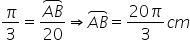So, the length of the minor arc of the chord is 20π/3 cm

Question 6: If in two circles, arcs of the same length subtend angles 60° and 75° at the centre, find the ratio of their radii.

Answer 6: Let the radii of the two circles be r1 and r2. Let an arc of length l subtend an angle of 60° at the centre of the circle of radius r1, and let an arc of length l subtend an angle of 75° at the centre of the other circle of radius r2.

We know that in a circle of radius r unit, if an arc of length l unit subtends an angle θ

θ =1/r or l=rθ

∴l=r1π /3 and l=r25π /12

⇒ r1π /3= r25π /12

⇒r1= r25 /4

⇒r1/r2=5/4

Thus, the ratio of the radii is 5:4.

Question 7: Find the angle in radian though which a pendulum swings if its length is 75 cm and the tip describes an arc of length

(i) 10 cm (ii) 15 cm (iii) 21 cm

Answer 7: We know that in a circle of radius r unit, if an arc of length l unit subtends

an angle θ radian at the centre, then θ =l/r

It is given that r = 75 cm

(i) Here, l = 10 cm